Open in App
Not now

# Class 10 NCERT Solutions- Chapter 6 Triangles – Exercise 6.3 | Set 1

• Last Updated : 18 Mar, 2021

### Question 1. State which pairs of triangles in Figure, are similar. Write the similarity criterion used by you for answering the question and also write the pairs of similar triangles in the symbolic form: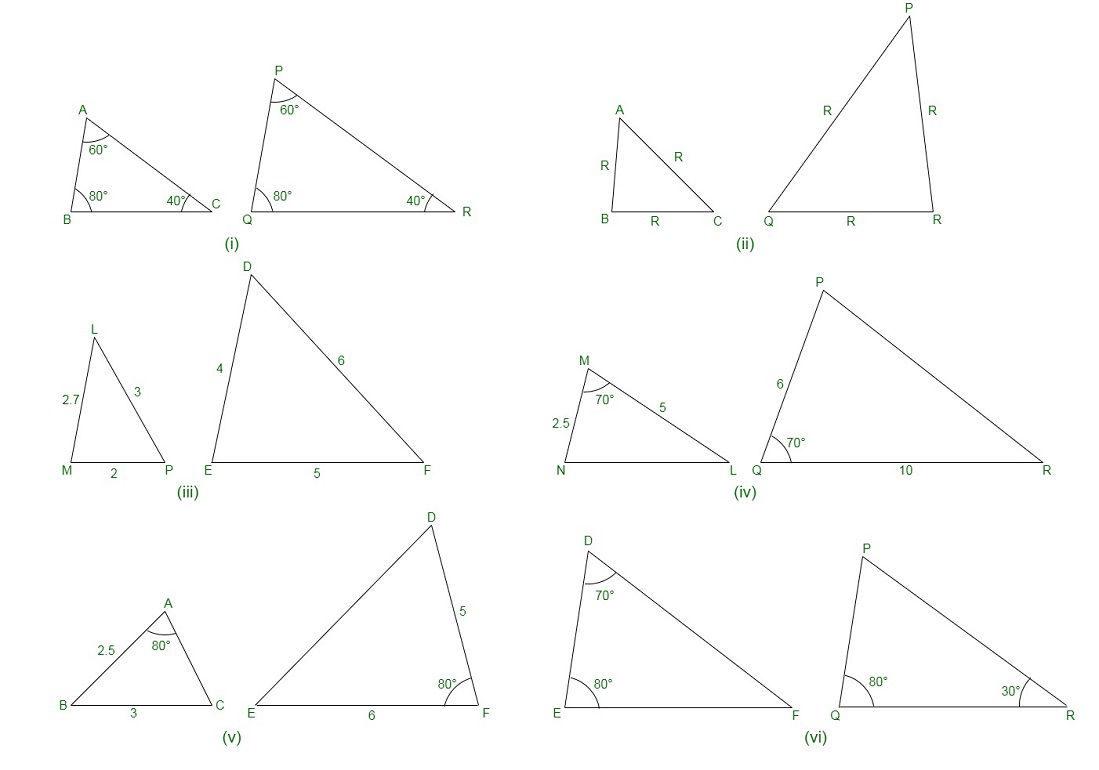Solution:

(i) We have, in ΔABC and ΔPQR,

∠A = ∠P = 60°

∠B = ∠Q = 80°

∠C = ∠R = 40°

By AAA similarity criterion, we have

∴ ΔABC ~ ΔPQR

(ii) We have, in ΔABC and ΔPQR,

Computing the ratios of corresponding sides,

AB/QR = 2.5/5 = 1/2

BC/RP = 2/4 = 1/2

CA/PQ = 3/6 = 1/2

AB/QR = BC/RP = CA/PQ

Now,

By SSS similarity criterion, we have

ΔABC ~ ΔQRP

(iii) We have, in ΔLMP and ΔDEF,

Now,

LM = 2.7, MP = 2, LP = 3, EF = 5, DE = 4, DF = 6

Computing the ratios of the corresponding sides,

MP/DE = 2/4 = 1/2

PL/DF = 3/6 = 1/2

LM/EF = 2.7/5 = 27/50

Since, MP/DE = PL/DF ≠ LM/EF

Therefore, ΔLMP and ΔDEF are not similar.

(iv) In ΔMNL and ΔQPR, it is given,

Computing the ratios of the corresponding sides,

MN/QP = ML/QR = 1/2

And,

∠M = ∠Q = 70°

By SAS similarity criterion

ΔMNL ~ ΔQPR

(v) In ΔABC and ΔDEF, we have,

AB = 2.5, BC = 3, ∠A = 80°, EF = 6, DF = 5, ∠F = 80°

Computing the ratios of the corresponding sides,

AB/DF = 2.5/5 = 1/2

And, BC/EF = 3/6 = 1/2

⇒ ∠B ≠ ∠F

Therefore, ΔABC and ΔDEF are not similar.

(vi) In ΔDEF,

By angle sum property of a triangle,

∠D + ∠E + ∠F = 180°

⇒ 70° + 80° + ∠F = 180°

⇒ ∠F = 180° – 70° – 80°

⇒ ∠F = 30°

Similarly, In ΔPQR,

By angle sum property of a triangle,

∠P + ∠Q + ∠R = 180

⇒ ∠P + 80° + 30° = 180°

⇒ ∠P = 180° – 80° -30°

⇒ ∠P = 70°

Now, in ΔDEF and ΔPQR, we have

∠D = ∠P = 70° [equal corresponding angles]

∠F = ∠Q = 80°

∠F = ∠R = 30°

Therefore,

By AAA similarity criterion,

ΔDEF ~ ΔPQR

### Question 2.  In the figure, ΔODC ∝ ¼ ΔOBA, ∠ BOC = 125° and ∠ CDO = 70°. Find ∠ DOC, ∠ DCO, and ∠ OAB.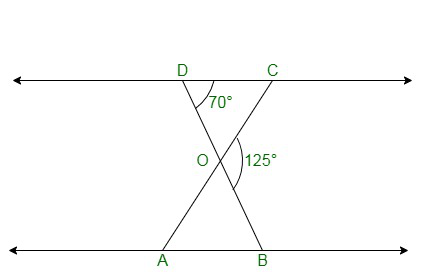Solution:

We know, DOB is a straight line, as given.

Therefore, ∠DOC + ∠COB = 180°

Also,

∠BOC = 125°

⇒ ∠DOC = 180° – 125°

= 55°

In ΔDOC

By angle sum property of a triangle,

∠DCO + ∠CDO + ∠DOC = 180°

∠CDO = 70°

⇒ ∠DCO + 70º + 55º = 180°

⇒ ∠DCO = 55°

Also, given,

ΔODC ∝ ¼ ΔOBA,

Therefore, ΔODC ~ ΔOBA.

Since, we know that the corresponding angles are equal in similar triangles,

Therefore, ∠OAB = ∠OCD

⇒ ∠ OAB = 55°

∠OAB = ∠OCD

∠OAB = 55°

### Question 3. Diagonals AC and BD of a trapezium ABCD with AB || DC intersect each other at the point O. Using a similarity criterion for two triangles, show that AO/OC = OB/OD

Solution: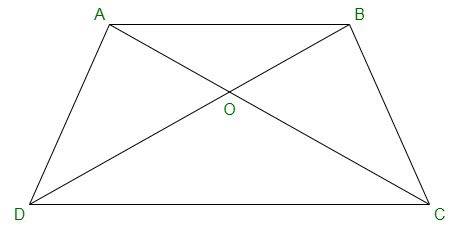In ΔDOC and ΔBOA,

Since, AB || CD, therefore the alternate interior angles are equal,

That is, ∠CDO = ∠ABO ….(i)

And,

∠DCO = ∠BAO …(ii)

Also, vertically opposite angles are equal;

That is,∠DOC = ∠BOA…(iii)

Hence, by AAA similarity criterion,

ΔDOC ~ ΔBOA [From eq(i) , (ii) and (iii)]

Since the triangles are similar, therefore the corresponding sides are proportional.

DO/BO = OC/OA

⇒OA/OC = OB/OD

Hence, proved.

### Question 4. In the fig., QR/QS = QT/PR and ∠1 = ∠2. Show that ΔPQS ~ ΔTQR.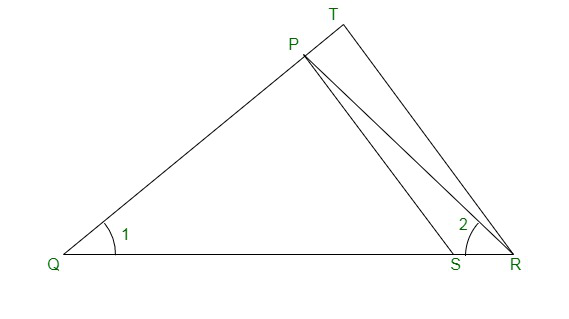Solution:

We have,

In ΔPQR,

∠PQR = ∠PRQ

That is, PQ = PR .…(i)

Given,

QR/QS = QT/PR

By using eq(i), we obtain,

QR/QS = QT/QP …….(ii)

In ΔPQS and ΔTQR,

QR/QS = QT/QP [By using eq(ii)]

∠Q = ∠Q

By SAS similarity criterion, therefore,

ΔPQS ~ ΔTQR

### Question 5. S and T are point on sides PR and QR of ΔPQR such that ∠P = ∠RTS. Show that ΔRPQ ~ ΔRTS.

Solution:

We know,

S and T are the points on sides PR and QR of ΔPQR.

And, ∠P = ∠RTS.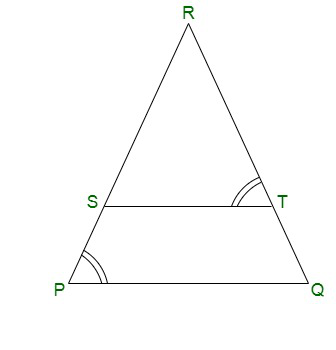In ΔRPQ and ΔRTS,

Given,

∠RTS = ∠QPS

∠R = ∠R (Common angle)

By AAA similarity criterion,

∴ ΔRPQ ~ ΔRTS

### Question 6. In the figure, if ΔABE ≅ ΔACD, show that ΔADE ~ ΔABC.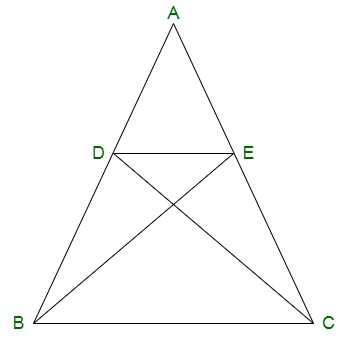Solution:

Given, ΔABE ≅ ΔACD.

By CPCT, we have

∴ AB = AC ……….(i)

On dividing the eq.(ii) by eq(i), we obtain,

Also, by common angle,

∠A = ∠A

By SAS similarity criterion, we have,

### Question 7. In the figure, altitudes AD and CE of ΔABC intersect each other at the point, P. Show, that: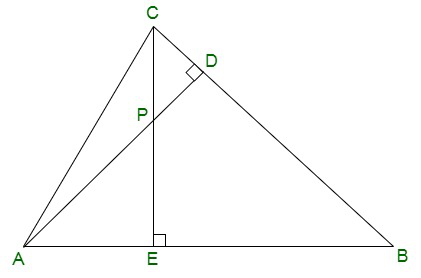(i) ΔAEP ~ ΔCDP

(ii) ΔABD ~ ΔCBE

(iv) ΔPDC ~ ΔBEC

Solution:

Given, altitudes AD and CE of ΔABC intersect each other at the point P.

(i) In ΔAEP and ΔCDP,

Since, both of the angles are 90° each.

∠AEP = ∠CDP

Since,

∠APE = ∠CPD (Vertically opposite angles)

By AA similarity criterion, we have,

ΔAEP ~ ΔCDP

(ii) In ΔABD and ΔCBE,

Since, both of the angles are 90° each.

∠ABD = ∠CBE (Common Angles)

By AA similarity criterion, we have,

ΔABD ~ ΔCBE

Since, both of the angles are 90° each.

∠PAE = ∠DAB (Common angles)

By AA similarity criterion, we have,

(iv) In ΔPDC and ΔBEC,

Since, both of the angles are 90° each.

∠PDC = ∠BEC

∠PCD = ∠BCE (Common angles)

By AA similarity criterion, we have,

ΔPDC ~ ΔBEC

### Question 8. E is a point on the side AD produced of a parallelogram ABCD and BE intersects CD at F. Show that ΔABE ~ ΔCFB.

Solution:

Given,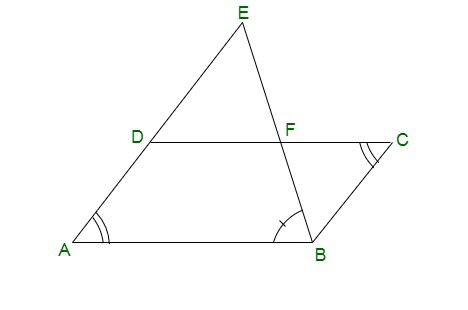E is a point on the side AD produced of a parallelogram ABCD and BE intersects CD at F.

Now,

In ΔABE and ΔCFB,

Since, the opposite angles of a parallelogram are equal, we have,

∠A = ∠C

Also, since AE || BC

∠AEB = ∠CBF (Alternate interior angles)

By AA similarity criterion,

∴ ΔABE ~ ΔCFB

### Question 9. In the figure, ABC and AMP are two right triangles, right-angled at B and M respectively, prove that: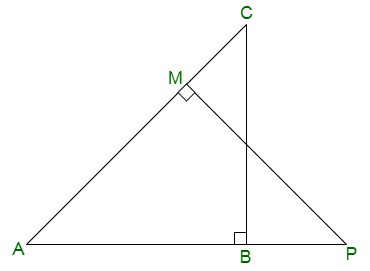(i) ΔABC ~ ΔAMP

(ii) CA/PA = BC/MP

Solution:

Given,

ABC and AMP are two right triangles, right-angled at B and M respectively.

(i) In ΔABC and ΔAMP, we have,

∠CAB = ∠MAP (common angles)

Since, both of the angles are equivalent to 90°

∠ABC = ∠AMP

By AA similarity criterion, we have,

∴ ΔABC ~ ΔAMP

(ii) As, ΔABC ~ ΔAMP (AA similarity criterion) [proved in part (i)]

Therefore, we know,

If two triangles are similar then the corresponding sides are always equal,

Hence, CA/PA = BC/MP

### Question 10. CD and GH are respectively the bisectors of ∠ACB and ∠EGF such that D and H lie on sides AB and FE of ΔABC and ΔEFG respectively. If ΔABC ~ ΔFEG, Show that:

(i) CD/GH = AC/FG

(ii) ΔDCB ~ ΔHGE

(iii) ΔDCA ~ ΔHGF

Solution:

Given,

CD and GH are respectively the bisectors of ∠ACB and ∠EGF such that D and H lie on sides AB and FE of ΔABC and ΔEFG respectively.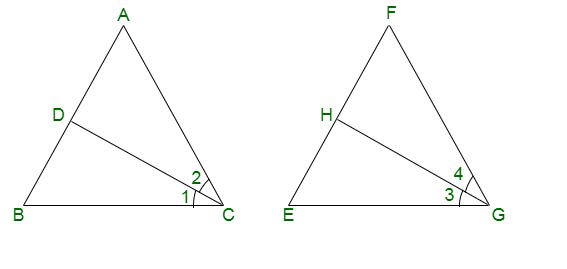(i) We have,

ΔABC ~ ΔFEG.

Therefore,

∠A = ∠F, ∠B = ∠E, and ∠ACB = ∠FGE

Now, since,

∠ACB = ∠FGE

∴ ∠ACD = ∠FGH (Angle bisector)

And, ∠DCB = ∠HGE

In ΔACD and ΔFGH,

∠A = ∠F

∠ACD = ∠FGH

By AA similarity criterion, we have,

∴ ΔACD ~ ΔFGH

⇒CD/GH = AC/FG

(ii) In ΔDCB and ΔHGE,

Proved in part (i)

∠DCB = ∠HGE

∠B = ∠E

By AA similarity criterion,

∴ ΔDCB ~ ΔHGE

(iii) In ΔDCA and ΔHGF,

∠ACD = ∠FGH

∠A = ∠F

By AA similarity criterion,

∴ ΔDCA ~ ΔHGF

My Personal Notes arrow_drop_up
Related Articles